JEE  >  VITEEE Maths Test - 3

# VITEEE Maths Test - 3

Test Description

## 40 Questions MCQ Test VITEEE: Subject Wise and Full Length MOCK Tests | VITEEE Maths Test - 3

VITEEE Maths Test - 3 for JEE 2023 is part of VITEEE: Subject Wise and Full Length MOCK Tests preparation. The VITEEE Maths Test - 3 questions and answers have been prepared according to the JEE exam syllabus.The VITEEE Maths Test - 3 MCQs are made for JEE 2023 Exam. Find important definitions, questions, notes, meanings, examples, exercises, MCQs and online tests for VITEEE Maths Test - 3 below.
Solutions of VITEEE Maths Test - 3 questions in English are available as part of our VITEEE: Subject Wise and Full Length MOCK Tests for JEE & VITEEE Maths Test - 3 solutions in Hindi for VITEEE: Subject Wise and Full Length MOCK Tests course. Download more important topics, notes, lectures and mock test series for JEE Exam by signing up for free. Attempt VITEEE Maths Test - 3 | 40 questions in 50 minutes | Mock test for JEE preparation | Free important questions MCQ to study VITEEE: Subject Wise and Full Length MOCK Tests for JEE Exam | Download free PDF with solutions
 1 Crore+ students have signed up on EduRev. Have you?
VITEEE Maths Test - 3 - Question 1

### The abcissa of the points of the curve y = x(x - 2)(x - 4) where tangents are parallel to x-axis is obtained as

Detailed Solution for VITEEE Maths Test - 3 - Question 1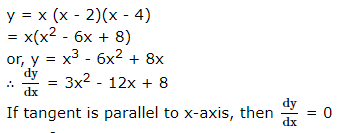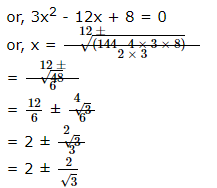VITEEE Maths Test - 3 - Question 2

### AOB is the positive quadrant of the ellipse x2 ∕ a2 + y2 ∕ b2 = 1, where OA = a, OB = b. The area between the arc AB and the chord AB of the ellipse is

VITEEE Maths Test - 3 - Question 3

### Let z₁ and z₂ be two roots of the equation z2 + az + b = 0, z being complex further, assume that the origin, z₁ and z₂ form an equilateral triangle, then

Detailed Solution for VITEEE Maths Test - 3 - Question 3
As z1, z2 are roots of z^2 + az + b
z1 + z2 = –a and z1 z2 = b
Again 0, z1,  z2 are vertices of an equilateral triangle.
Therefore, 0^2 + z1^2 + z2^2 = 0z1 + z1z2 + 0z2 = 0
z1^2 + z2^2 = z1z2
(z1 + z2)^2 = 3z1z2
The correct option is B.
VITEEE Maths Test - 3 - Question 4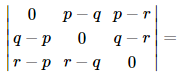VITEEE Maths Test - 3 - Question 5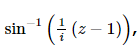where z is non real, can be the angle of a triangle if

VITEEE Maths Test - 3 - Question 6

If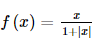for x ∈ ℝ then f ′ 0 =

VITEEE Maths Test - 3 - Question 7

The value of f(0) so that f x = sin x/x is continuous at x = 0 is

VITEEE Maths Test - 3 - Question 8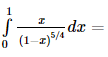VITEEE Maths Test - 3 - Question 9

If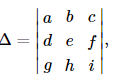and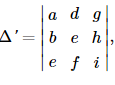then

VITEEE Maths Test - 3 - Question 10

The solution of differential equation (xdy/dx)=y+x2 is

VITEEE Maths Test - 3 - Question 11

Solution of (x+y-1) dx+(2x+2y-3) dy = 0 is

VITEEE Maths Test - 3 - Question 12

The general solution of the equation x2(dy/dx)=2 is

VITEEE Maths Test - 3 - Question 13

The general solution of the differential equation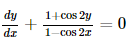is given by

VITEEE Maths Test - 3 - Question 14

If y=x2(x-2)2, then the values of x for which y is increasing, are

VITEEE Maths Test - 3 - Question 15

If S is the set of all real x such that 2x - 1/2x3 + 3x2 + x is positive, then S contains

VITEEE Maths Test - 3 - Question 16

∫tan-1x dx =

VITEEE Maths Test - 3 - Question 17

∫[(1)/(x+xlogx)]dx=

VITEEE Maths Test - 3 - Question 18

sec-1[sec(-300)]=

VITEEE Maths Test - 3 - Question 19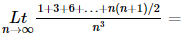Detailed Solution for VITEEE Maths Test - 3 - Question 19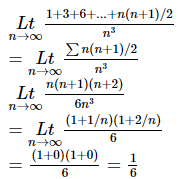VITEEE Maths Test - 3 - Question 20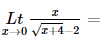VITEEE Maths Test - 3 - Question 21

The dual of the statement [ p ∨ ∼ q ] ∧ ∼ p is

VITEEE Maths Test - 3 - Question 22

If A,B,C are matrices of order nxn then (ABC)'=

VITEEE Maths Test - 3 - Question 23

If A2 = 2A − I then for n ≠ 2, An =

VITEEE Maths Test - 3 - Question 24

Out of 15 points in a plane, no three are in a straight line except 8 points which are collinear. How many triangles can be formed by joining them?

Detailed Solution for VITEEE Maths Test - 3 - Question 24

Solution :-  No. of Δs = 15C3 - 8C3

= 15!/(3!*12!) - (8!/(3!*5!)

= 455 - 56 = 399

VITEEE Maths Test - 3 - Question 25

Let A and B be two events such that P(A) = 0.3, P(A∪B) = 0.8, if A and B are independent events, then P(B) is equal to

VITEEE Maths Test - 3 - Question 26

The probability that a man can hit a target is 3/4. He tries 5 times. The probability that he will hit the target at least three times is

VITEEE Maths Test - 3 - Question 27

In two events P(A∪B) = 5/6, P(A) = 5/6, P(B) = 2/3 then A and B are

VITEEE Maths Test - 3 - Question 28

In a ΔABC , a = 8 , b = 10 and c = 12. Then C is equal to

Detailed Solution for VITEEE Maths Test - 3 - Question 28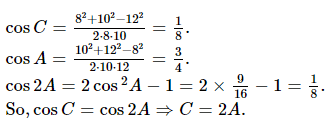VITEEE Maths Test - 3 - Question 29

If a, b, c of a triangle are in A.P., then cot C/2 =

VITEEE Maths Test - 3 - Question 30

The most stable measure of central tendency is

VITEEE Maths Test - 3 - Question 31

A group of 10 items has mean 6. If the mean of 4 of these items is 7.5, then the mean of the remaining items is

VITEEE Maths Test - 3 - Question 32

If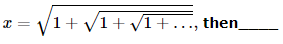Detailed Solution for VITEEE Maths Test - 3 - Question 32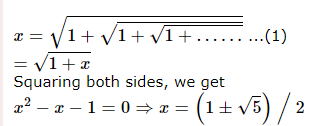From (1), x must be greater than 1 (taking + ve sign.)
∴ 1 < x < 2

VITEEE Maths Test - 3 - Question 33

If the roots of the equation x2+2mx+m2-2m+6=0 are equal, then the value of m is

VITEEE Maths Test - 3 - Question 34

If a line makes angles α,β,γ with the +ve direction of x,y and z axis respectively, then cos2α+cos2β+cos2γ

VITEEE Maths Test - 3 - Question 35

If the direction cosines of a line are < (1/c), (1/c), (1/c) >, then

VITEEE Maths Test - 3 - Question 36

The general value of θ which satisfies the equations sinθ=-(1/2) and tanθ=(1/√3) is

VITEEE Maths Test - 3 - Question 37

If a, b, c are in A.P. then the straight line ax + by + c = 0 will always pass through a fixed point whose coordinates are

VITEEE Maths Test - 3 - Question 38

The equation of the circle having its centre on the line x + 2y - 3 = 0 and passing through the points of intersection of the circles x2+ y2 - 2x - 4y + 1 = 0 and x2 + y2 - 4x - 2y + 4 = 0 is

VITEEE Maths Test - 3 - Question 39

If a=i+j-k, b=-i+2j+k and c=-i+2j-k, the unit vector perpendicular to a+b and b+c is

VITEEE Maths Test - 3 - Question 40

Let p=(x+4y)a+(2x+y+1)b and q=(y-2x+2)a+(2x-3y-1)b, where a and b are non-coplanar vectors. If 3p=2q, then the value of x and y are

## VITEEE: Subject Wise and Full Length MOCK Tests

1 videos|3 docs|73 tests
 Use Code STAYHOME200 and get INR 200 additional OFF Use Coupon Code
Information about VITEEE Maths Test - 3 Page
In this test you can find the Exam questions for VITEEE Maths Test - 3 solved & explained in the simplest way possible. Besides giving Questions and answers for VITEEE Maths Test - 3, EduRev gives you an ample number of Online tests for practice

## VITEEE: Subject Wise and Full Length MOCK Tests

1 videos|3 docs|73 tests